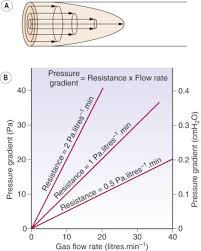## How to Calculate and Solve for Gas Flow of Reservoir Fluid Flow | The Calculator EncyclopediaThe image above represents gas flow.

To compute for the gas flow, four essential parameters are needed and these parameters are Performance Co-efficient (C), Average Reservoir Pressure (Pr*), Flowing Bore Hole Pressure (Pwf*) and Exponent (n).

The formula for calculating the gas flow:

Qg = C(Pr*² – Pwf*²)n

Where;

Qg = Gas Flow
C = Performance Co-efficient
Pr* = Average Reservoir Pressure
Pwf* = Flowing Bore Hole Pressure
n = Exponent

Let’s solve an example;
Find the gas flow when a performance Co-efficient is 9, average reservoir pressure is 10, flowing bore hole pressure is 5 and an exponent of 20 is given.

This implies that;

C = Performance Co-efficient = 9
Pr* = Average Reservoir Pressure = 10
Pwf* = Flowing Bore Hole Pressure = 5
n = Exponent = 20

Qg = C(Pr*² – Pwf*²)n
Qg = 9(10² – 5²)20
Qg = 9(100 – 25)20
Qg = 9(75)20
Qg = 9(3.17e+37)
Qg = 2.85e+38

Therefore, the gas flow is 2.85e+38.